$\newcommand{\NN}{\mathbb{N}} \newcommand{\CC}{\mathbb{C}} \newcommand{\GG}{\mathbb{G}} \newcommand{\LL}{\mathbb{L}} \newcommand{\PP}{\mathbb{P}} \newcommand{\QQ}{\mathbb{Q}} \newcommand{\RR}{\mathbb{R}} \newcommand{\VV}{\mathbb{V}} \newcommand{\ZZ}{\mathbb{Z}} \newcommand{\FF}{\mathbb{F}} \newcommand{\KK}{\mathbb{K}} \newcommand{\UU}{\mathbb{U}} \newcommand{\EE}{\mathbb{E}} \newcommand{\Aa}{\mathcal{A}} \newcommand{\Bb}{\mathcal{B}} \newcommand{\Cc}{\mathcal{C}} \newcommand{\Dd}{\mathcal{D}} \newcommand{\Ee}{\mathcal{E}} \newcommand{\Ff}{\mathcal{F}} \newcommand{\Gg}{\mathcal{G}} \newcommand{\Hh}{\mathcal{H}} \newcommand{\Ii}{\mathcal{I}} \newcommand{\Jj}{\mathcal{J}} \newcommand{\Kk}{\mathcal{K}} \newcommand{\Ll}{\mathcal{L}} \newcommand{\Mm}{\mathcal{M}} \newcommand{\Nn}{\mathcal{N}} \newcommand{\Oo}{\mathcal{O}} \newcommand{\Pp}{\mathcal{P}} \newcommand{\Qq}{\mathcal{Q}} \newcommand{\Rr}{\mathcal{R}} \newcommand{\Ss}{\mathcal{S}} \newcommand{\Tt}{\mathcal{T}} \newcommand{\Uu}{\mathcal{U}} \newcommand{\Vv}{\mathcal{V}} \newcommand{\Ww}{\mathcal{W}} \newcommand{\Xx}{\mathcal{X}} \newcommand{\Yy}{\mathcal{Y}} \newcommand{\Zz}{\mathcal{Z}} \newcommand{\al}{\alpha} \newcommand{\la}{\lambda} \newcommand{\ga}{\gamma} \newcommand{\Ga}{\Gamma} \newcommand{\La}{\Lambda} \newcommand{\Si}{\Sigma} \newcommand{\si}{\sigma} \newcommand{\be}{\beta} \newcommand{\de}{\delta} \newcommand{\De}{\Delta} \renewcommand{\phi}{\varphi} \renewcommand{\th}{\theta} \newcommand{\om}{\omega} \newcommand{\Om}{\Omega} \renewcommand{\epsilon}{\varepsilon} \newcommand{\Calpha}{\mathrm{C}^\al} \newcommand{\Cbeta}{\mathrm{C}^\be} \newcommand{\Cal}{\text{C}^\al} \newcommand{\Cdeux}{\text{C}^{2}} \newcommand{\Cun}{\text{C}^{1}} \newcommand{\Calt}{\text{C}^{#1}} \newcommand{\lun}{\ell^1} \newcommand{\ldeux}{\ell^2} \newcommand{\linf}{\ell^\infty} \newcommand{\ldeuxj}{{\ldeux_j}} \newcommand{\Lun}{\text{\upshape L}^1} \newcommand{\Ldeux}{\text{\upshape L}^2} \newcommand{\Lp}{\text{\upshape L}^p} \newcommand{\Lq}{\text{\upshape L}^q} \newcommand{\Linf}{\text{\upshape L}^\infty} \newcommand{\lzero}{\ell^0} \newcommand{\lp}{\ell^p} \renewcommand{\d}{\ins{d}} \newcommand{\Grad}{\text{Grad}} \newcommand{\grad}{\text{grad}} \renewcommand{\div}{\text{div}} \newcommand{\diag}{\text{diag}} \newcommand{\pd}{ \frac{ \partial #1}{\partial #2} } \newcommand{\pdd}{ \frac{ \partial^2 #1}{\partial #2^2} } \newcommand{\dotp}{\langle #1,\,#2\rangle} \newcommand{\norm}{|\!| #1 |\!|} \newcommand{\normi}{\norm{#1}_{\infty}} \newcommand{\normu}{\norm{#1}_{1}} \newcommand{\normz}{\norm{#1}_{0}} \newcommand{\abs}{\vert #1 \vert} \newcommand{\argmin}{\text{argmin}} \newcommand{\argmax}{\text{argmax}} \newcommand{\uargmin}{\underset{#1}{\argmin}\;} \newcommand{\uargmax}{\underset{#1}{\argmax}\;} \newcommand{\umin}{\underset{#1}{\min}\;} \newcommand{\umax}{\underset{#1}{\max}\;} \newcommand{\pa}{\left( #1 \right)} \newcommand{\choice}{ \left\{ \begin{array}{l} #1 \end{array} \right. } \newcommand{\enscond}{ \left\{ #1 \;:\; #2 \right\} } \newcommand{\qandq}{ \quad \text{and} \quad } \newcommand{\qqandqq}{ \qquad \text{and} \qquad } \newcommand{\qifq}{ \quad \text{if} \quad } \newcommand{\qqifqq}{ \qquad \text{if} \qquad } \newcommand{\qwhereq}{ \quad \text{where} \quad } \newcommand{\qqwhereqq}{ \qquad \text{where} \qquad } \newcommand{\qwithq}{ \quad \text{with} \quad } \newcommand{\qqwithqq}{ \qquad \text{with} \qquad } \newcommand{\qforq}{ \quad \text{for} \quad } \newcommand{\qqforqq}{ \qquad \text{for} \qquad } \newcommand{\qqsinceqq}{ \qquad \text{since} \qquad } \newcommand{\qsinceq}{ \quad \text{since} \quad } \newcommand{\qarrq}{\quad\Longrightarrow\quad} \newcommand{\qqarrqq}{\quad\Longrightarrow\quad} \newcommand{\qiffq}{\quad\Longleftrightarrow\quad} \newcommand{\qqiffqq}{\qquad\Longleftrightarrow\qquad} \newcommand{\qsubjq}{ \quad \text{subject to} \quad } \newcommand{\qqsubjqq}{ \qquad \text{subject to} \qquad }$

Source Separation with Sparsity

# Source Separation with Sparsity

This numerical tour explore local Fourier analysis of sounds, and its application to source separation from stereo measurements.

## Installing toolboxes and setting up the path.

You need to download the following files: signal toolbox and general toolbox.

You need to unzip these toolboxes in your working directory, so that you have toolbox_signal and toolbox_general in your directory.

For Scilab user: you must replace the Matlab comment '%' by its Scilab counterpart '//'.

Recommandation: You should create a text file named for instance numericaltour.sce (in Scilab) or numericaltour.m (in Matlab) to write all the Scilab/Matlab command you want to execute. Then, simply run exec('numericaltour.sce'); (in Scilab) or numericaltour; (in Matlab) to run the commands.

Execute this line only if you are using Matlab.

getd = @(p)path(p,path); % scilab users must *not* execute this


Then you can add the toolboxes to the path.

getd('toolbox_signal/');
getd('toolbox_general/');


## Sound Mixing

We load 3 sounds and simulate a stero recording by performing a linear blending of the sounds.

For Scilab users, it is safer to extend the stack size. For Matlab users this does nothing.

extend_stack_size(4);


n = 1024*16;
s = 3; % number of sound
p = 2; % number of micros
options.subsampling = 1;
x = zeros(n,3);
% normalize the energy of the signals
x = x./repmat(std(x,1), [n 1]);


We mix the sound using a 2x3 transformation matrix. Here the direction are well-spaced, but you can try with more complicated mixing matrices.

% compute the mixing matrix
theta = linspace(0,pi(),s+1); theta(s+1) = [];
theta(1) = .2;
M = [cos(theta); sin(theta)];
% compute the mixed sources
y = x*M';


Display of the sounds and their mix.

clf;
for i=1:s
subplot(s,1,i);
plot(x(:,i)); axis('tight');
set_graphic_sizes([], 20);
title(strcat('Source #',num2str(i)));
end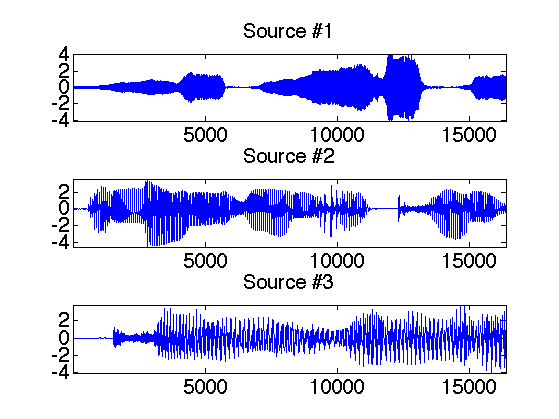Display of the micro output.

clf;
for i=1:p
subplot(p,1,i);
plot(y(:,i)); axis('tight');
set_graphic_sizes([], 20);
title(strcat('Micro #',num2str(i)));
end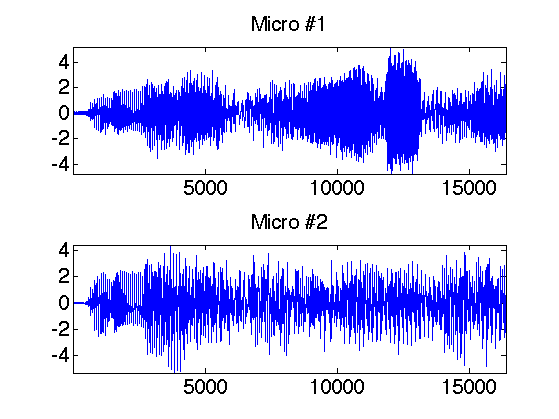## Local Fourier analysis of sound.

In order to perform the separation, one performs a local Fourier analysis of the sound. The hope is that the sources will be well-separated over the Fourier domain because the sources are sparse after a STFT.

First set up parameters for the STFT.

options.n = n;
w = 128;   % size of the window
q = w/4;    % overlap of the window


Compute the STFT of the sources.

clf; X = []; Y = [];
for i=1:s
X(:,:,i) = perform_stft(x(:,i),w,q, options);
subplot(s,1,i);
plot_spectrogram(X(:,:,i));
set_graphic_sizes([], 20);
title(strcat('Source #',num2str(i)));
end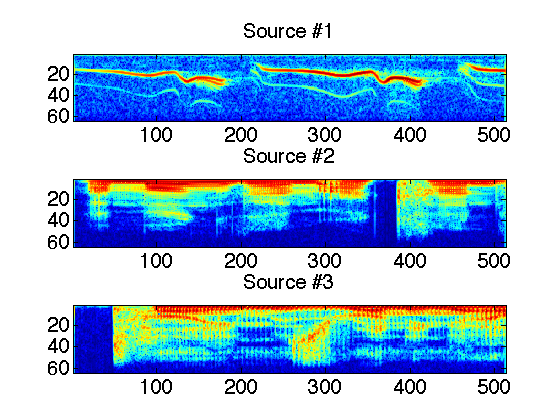Exercice 1: (check the solution) Compute the STFT of the micros, and store them into a matrix Y.

exo1;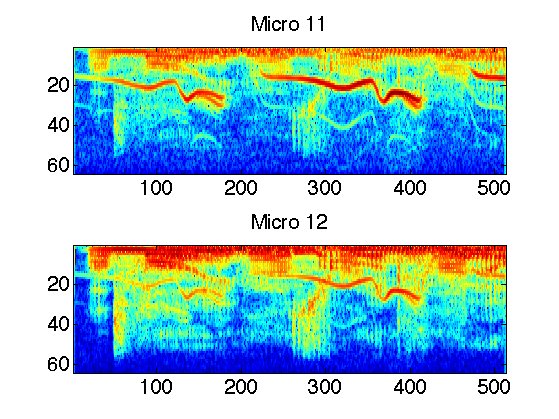## Estimation of Mixing Direction by Clustering

Since the sources are quite sparse over the Fourier plane, the directions are well estimated by looking as the direction emerging from a point clouds of the transformed coefficients.

First we compute the position of the point cloud.

mf = size(Y,1);
mt = size(Y,2);
P = reshape(Y, [mt*mf p]);
P = [real(P);imag(P)];


Then we keep only the 5% of points with largest energy.

Display some points in the original (spacial) domain.

% number of displayed points
npts = 6000;
% display original points
sel = randperm(n); sel = sel(1:npts);
clf;
plot(y(sel,1), y(sel,2), '.');
axis([-1 1 -1 1]*5);
set_graphic_sizes([], 20);
title('Time domain');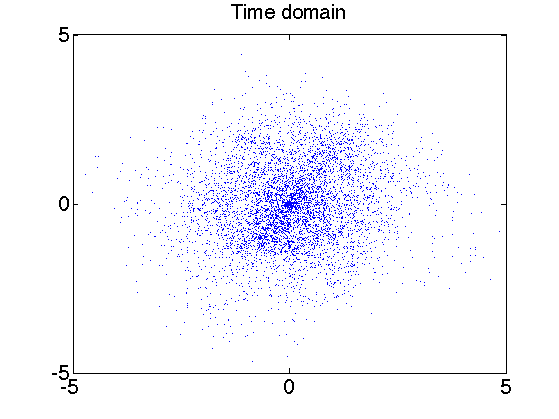Exercice 2: (check the solution) Display some points of P in the transformed (time/frequency) domain.

exo2;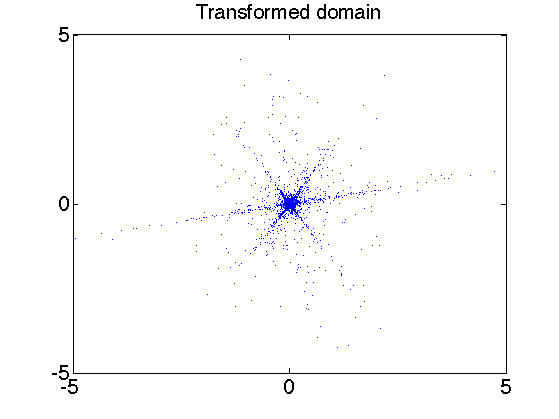We compute the angle associated to each point over the transformed domain. The histograms shows the main direction of mixing.

Theta = mod(atan2(P(:,2),P(:,1)), pi());
% display histograms
nbins = 100;
[h,t] = hist(Theta, nbins);
h = h/sum(h);
clf;
bar(t,h); axis('tight');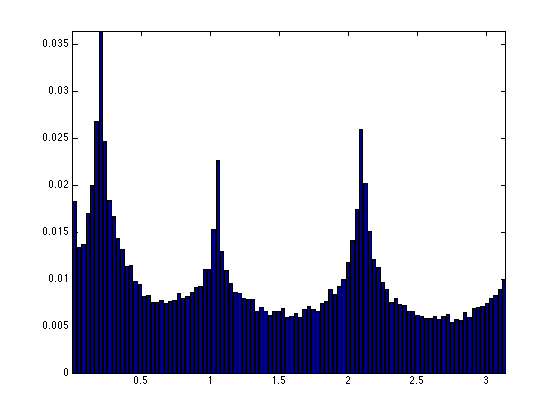Exercice 3: (check the solution) The histogram computed from the whole set of points are not peacked enough. To stabilize the detection of mixing direction, compute an histogram from a reduced set of point that have the largest amplitude.

exo3;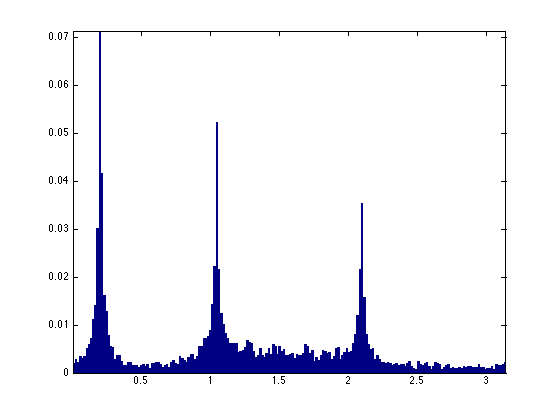Exercice 4: (check the solution) Detect the direction M1 approximating the true direction M by looking at the local maxima of the histogram. First detect the set of local maxima, and then keep only the three largest.

exo4;

M =

0.9801    0.5000   -0.5000
0.1987    0.8660    0.8660

M1 =

0.9803    0.5010   -0.5028
0.1973    0.8655    0.8644



## Separation of the Sources using Clustering

Once the mixing direction are known, one can project the sources on the direction.

We compute the projection of the coefficients Y on each estimated direction.

A = reshape(Y, [mt*mf p]);
% compute the projection of the coefficients on the directions
C = abs( M1'*A' );


At each point x, the index I(x) is the direction which creates the largest projection.

% I is the index of the closest source
[tmp,I] = compute_max(C, 1);
I = reshape(I, [mf mt]);


An additional denoising is achieved by removing small coefficients.

% do not take into account too small coefficients
T = .05;
D = sqrt(sum( abs(Y).^2, 3));
I = I .* (D>T);


We can display the segmentation of the time frequency plane.

clf;
imageplot(I(1:mf/2,:));
axis('normal');
set_colormap('jet');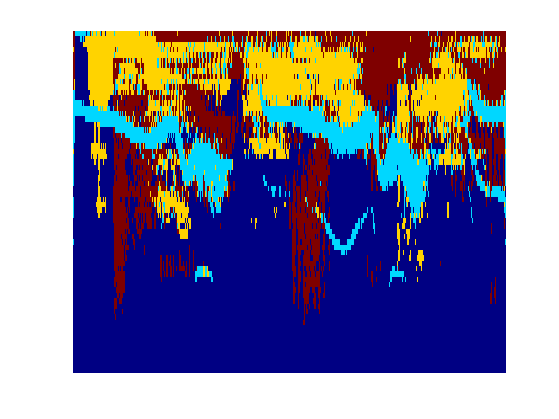The recovered coefficients are obtained by projection.

Proj = M1'*A';
Xr = [];
for i=1:s
Xr(:,:,i) = reshape(Proj(i,:), [mf mt]) .* (I==i);
end


The estimated signals are obtained by inverting the STFT.

for i=1:s
xr(:,i) = perform_stft(Xr(:,:,i),w,q, options);
end


One can display the recovered signals.

clf;
for i=1:s
subplot(s,1,i);
plot(xr(:,i)); axis('tight');
set_graphic_sizes([], 20);
title(strcat('Estimated source #',num2str(i)));
end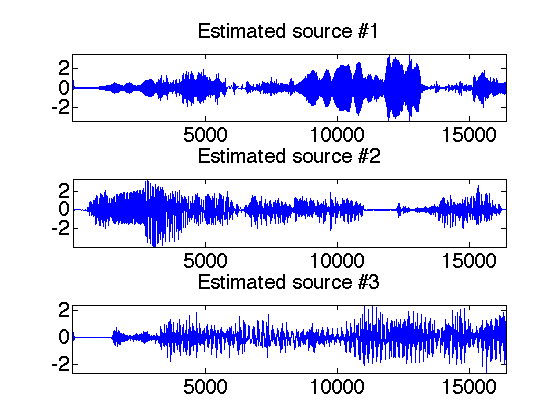One can listen to the recovered sources.

i = 1;
sound(x(:,i),fs);
sound(xr(:,i),fs);